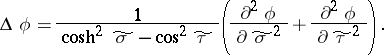# Elliptic coordinates

(diff) ← Older revision | Latest revision (diff) | Newer revision → (diff)

Two numbersandconnected with rectangular Cartesian coordinates by the formulas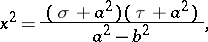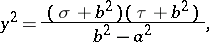where.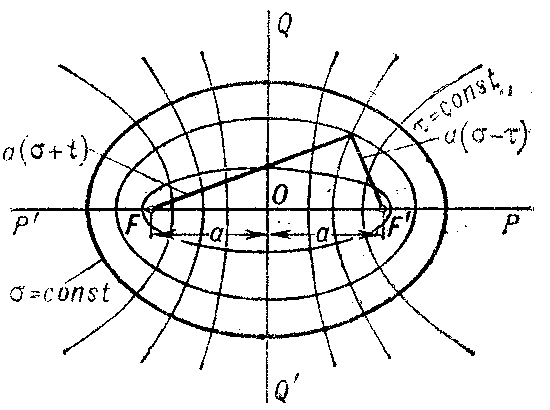Figure: e035440a

The coordinate lines are (see Fig.): confocal ellipses (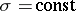) and hyperbolas () with foci () and (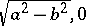). The system of elliptic coordinates is orthogonal. To every pair of numbersandcorrespond four points, one in each quadrant of the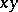-plane.

The Lamé coefficients are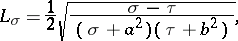In elliptic coordinates the Laplace equation allows separation of variables.

Degenerate elliptic coordinates are two numbers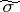and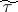connected withandby the formulas (for,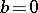):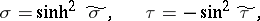and with Cartesian coordinatesandbywhere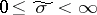and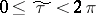. Occasionally these coordinates are also called elliptic.

The Lamé coefficients are: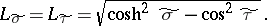The area element is:The Laplace operator is: